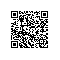# 简单选择排序

### 基本思想：

(1)设待排序的记录存放在数组r[1…n ]中，第一趟从r开始，通过n-1次比较，从n个记录中选出关键字最小的记录，记为r[k]，交换r和r[k].

(2)第二趟从r开始，通过n-2次比较，从n-1个记录中选出关键字最小的记录，记为r[k],交换r和r[k]。

(3)第i趟从r[i]开始，通过n-i次比较，从n-1+1个记录中选出关键字最小的记录，记为r[k]，交换r[i]和r[k].

(4)经过n-1趟，排序完成。void SelectSort(SqList &K)
{
//对顺序表L做简单选择排序
for(i=1;i<L.length;++i)
{
//在L.r[i...L.length]中选择关键字最小的记录
k=i;
for(j=j+1;j<=L.length;j++)
if(L.r[j].key<L.r[k].key)k=j;//k指向此趟排序中关键字最小的记录
if(k!=i)
{
t=L.r[i];
L.r[i]=L.r[k];//交换r[i]与r[k]
L.r[k]=t;
}
}
}


• 最好情况： 0

• 最坏情况：3(n-1)

### 算法特点

            不稳定使用钉钉扫一扫加入圈子
+ 订阅